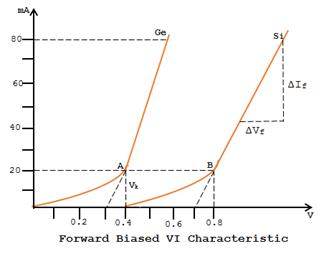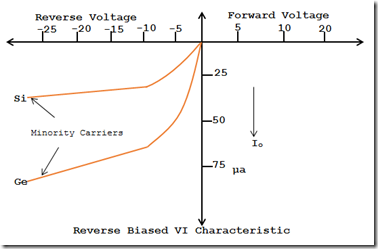# VI Characteristics of PN Junction

### PN Junction

Voltage Ampere or VI characteristics of a PN junction are the curve between voltage across the junction and the circuit current. Usually, voltage is taken along X-axis and current along Y-axis.

Figure below shows that the circuit diagram to determine the VI characteristic of a PN junction.### Forward VI Characteristic:

• A typical VI characteristic for a forward biased PN junction is shown in the figure below.
• It is seen that the forward current rises exponentially with the applied forward voltage. However, at ordinary room temperature, a potential difference of about 0.3V is required before a reasonable amount of forward current starts flowing in a germanium junction.
• This voltage is known as threshold voltage Vth  (OR)  CUT IN VOLTAGE  (OR)  KNEE VOLTAGE Vk. Its value for silicon junction is about 0.7 volt.### Reverse Biased VI Characteristic:

The figure below shows that the VI characteristic of a reverse biased PN junction. It is seen that as reverse voltage is increases from zero; the reverse current quickly rises to its maximum or saturation value.### Combined Forward and Reverse Biased PN Junction:

• The figure below shows that the combined forward and reverse biased VI characteristics for both Ge and Si are shown in the figure.
• It is seen that leakage current of Ge junction is much more than that of Si junction.Important Terms:
Two important terms often used with PN junction are breakdown voltage and knee voltage.

Breakdown Voltage:
It is the minimum reverse voltage at which PN junction breaks down with sudden rise in reverse current.

Knee Voltage:
It is the forward voltage at which the current through PN junction starts to increase rapidly.

Peak Inverse voltage:
It is the maximum reverse voltage that can be applied to the PN junctions without damage to the junction. If the reverse voltage across the junction exceeds its PIV, the junction may be destroyed due to excessive heat.0 CommentsComments